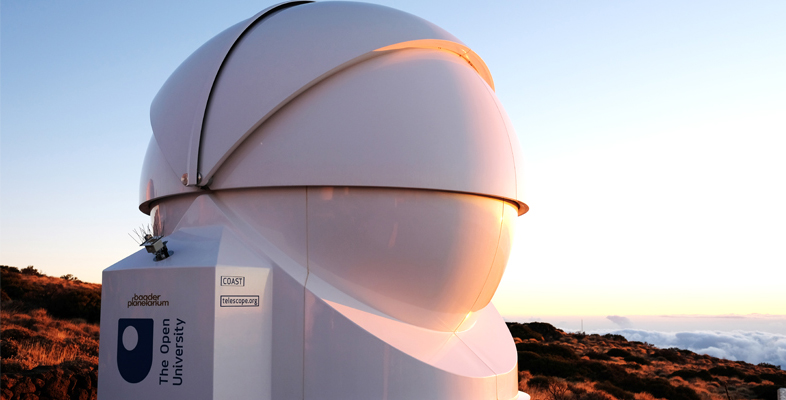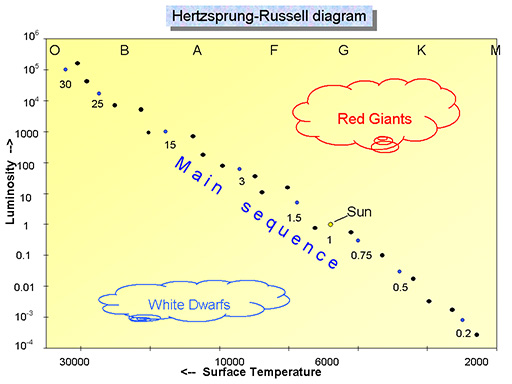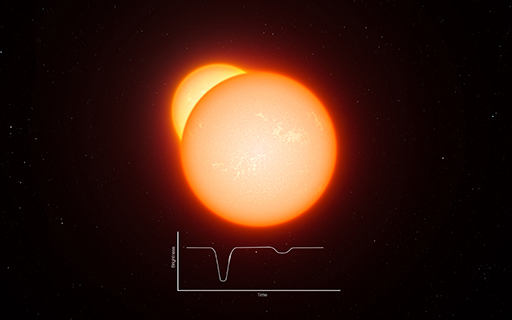Science, Maths & Technology

### Become an OU studentAstronomy with an online telescope

Start this free course now. Just create an account and sign in. Enrol and complete the course for a free statement of participation or digital badge if available.

# 3.1 Measuring the masses of stars

In Week 5 you learned that the Sun has a mass of . To avoid having to work with such huge numbers and make it easier to compare the masses of stars, we can consider their masses as multiples of the Sun’s mass (the solar mass). On this scale, the Sun would have a mass of 1 solar mass, and a star with ten times the mass of the Sun would be 10 solar masses, and so on.Figure 4 The HR diagram, showing the mass of stars along the main sequence as multiples of the Sun’s mass. The letters at the top indicate the spectral class of the stars, with O and B representing the brightest and hottest stars, and K and M at the other end representing the faintest and reddest stars.

Full resolution image here [Tip: hold Ctrl and click a link to open it in a new tab. (Hide tip)]

Here is the HR diagram again, this time with numbers indicating the masses of selected stars along the main sequence as multiples of the Sun’s mass. These range from small red K and M stars, much smaller than the Sun, to hot luminous O and B stars up to 30 times more massive than the Sun.

How do we know the masses of these stars? You have already seen how the colour (and hence temperature) of a star can be measured from the shape of its spectrum, and how the luminosities of stars can be compared and measured using stars in a cluster to eliminate the distance factor. Both of these techniques have involved a certain degree of ingenuity in addition to straightforward measurement. To find the masses of stars requires an equal amount of ingenuity and thought.

In Section 2.3 of Week 5 you saw how you can work out the mass of the Sun by measuring the size and period of the orbit of a planet such as Jupiter and applying Newton’s laws of gravity. One technique for determining the masses of stars uses a very similar principle, together with the fact that many stars occur as binary stars – two stars orbiting one another. Given that stars often form in clusters from a cloud of collapsing gas, this is actually relatively common, as many stars are formed close to one another at the same time, and some of these will pair up to form binary systems.Figure 5 Artist’s impression of eclipsing binary stars. The light curve below shows how the brightness as seen from Earth dips on a regular basis as one star passes in front of another. (ESO.org)

You will learn a lot more about binary stars next week, but for now the important point is that it is possible to measure the period of orbit for some of these stars around one another, and in doing so to find their masses in the same way that we did for the Sun, using the period of Jupiter’s orbit. Figure 5 shows one such type of binary system, where one star passes in front of another, causing a drop in the light seen from Earth. The time between these dips tells us the period of the orbit; from this the masses of the stars can be calculated. Of course, not all stars are binaries, but it turns out that binary stars fit on the main sequence exactly alongside single stars and so there is every reason to suppose that their masses will be typical of stars of the same spectral type.